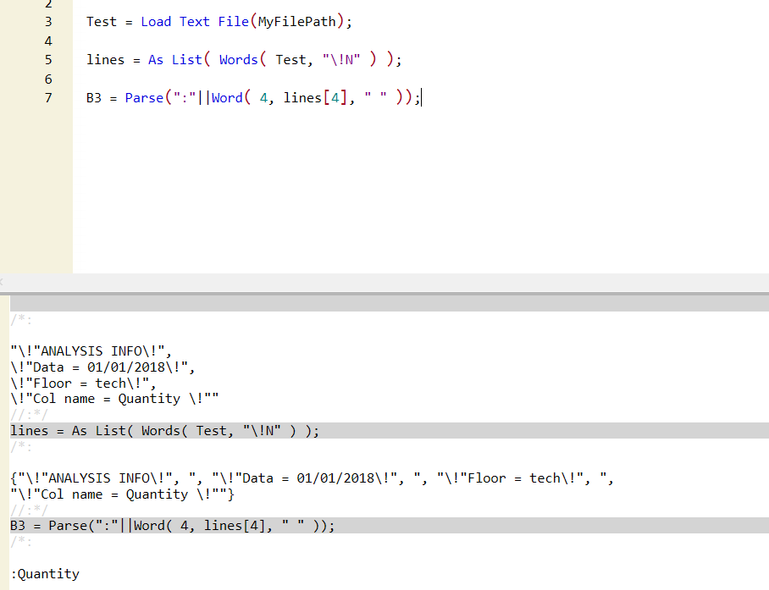Choose Language Hide Translation Bar
Highlighted

## Error with Surviving plot and use of Parse()

Hi all,

I hope someone can help me in understanding why my script doesn't work properly.

I have a txt file with some information stored in. One of them is the name of a column that I want to use for the elaboration of other tables.

``````textfile = Load Text File( path_elab || "\Analysis_info.txt" );
lines = Words( textfile, "\!N" );B3 = Parse( ":" || Word( 4, lines, " " ) );``````

B3 stores the column I want to use trough the script.

The script works and makes plots, till it arrives at :

``````	dt_plot = dt_stack << Survival(
Y( :A  ),
Censor( :C),
Grouping( B3 ),
Failure Plot( 1 ),
Survival Plot( 0 ),
Censor Code( 0 ),
By( :Z )
);``````

In this case the script stops, reporting Bad Argument( {B3} ) .

The fact is that if I comment the line containing By (:Z), the script works.

Do you know why this happens?

Thank you!

1 ACCEPTED SOLUTION

Accepted Solutions
Highlightedstan_koprowskiCommunity Manager

## Re: Error with Surviving plot and use of Parse()

Hi @Domix,

You are close with what you have written.

If you wrap B3 with As Column it should work.

``````Names Default To Here( 1 );
dt = Open( "\$SAMPLE_DATA/Rats.jmp" );
//Change names to work with example provided
:Group << Set Name( "Quantity" );
:days << Set Name( "A" );
:Censor << Set Name( "C" );

lines = {"ANALYSIS INFO", "Data = 01/01/2018",
"Floor = tech", "Col name = Quantity "};
B3 = Parse( ":" || Word( 4, lines, " " ) );

//B3 is a column //I commented out the 'by' variable as I did not have one in the sample data
Survival(
Y( :A ),
Censor( :C ),
Grouping( As Column( B3 ) ),
Failure Plot( 1 ),
Survival Plot( 0 ),
Censor Code( 0 )//,
//By( :Z )
);``````

cheers,

Stan

8 REPLIES 8
Highlighted

## Re: Error with Surviving plot and use of Parse()

@Domix,
Can you post a copy of the log that shows the result of these lines and the error message ?

Best
Uday
Highlighted

## Re: Error with Surviving plot and use of Parse()

Hi Uday,

here the log

``````Not Found in access or evaluation of 'Survival' , Bad Argument( {B3} ), Survival(/*###*/Y( :A ),
Censor( :C),
Grouping( B3 ),
Failure Plot( 1 ),
Survival Plot( 0 ),
Censor Code( 0 ),
By( :Z)
)

``````
Highlighted

## Re: Error with Surviving plot and use of Parse()

@Domix,
Please show what is contained in B3 as well. In your response, kindly show the log for all the lines of code you pasted not just the error.

Best
Uday
Highlighted

## Re: Error with Surviving plot and use of Parse()

``````lines = {"ANALYSIS INFO", "Data = 01/01/2018", "Floor = tech", "Col name = Quantity "}

B3 = Parse(":"||Word( 4, lines, " " ));``````

B3 should be :Quantity, if I go on it with the pointer, but if I run just B3 is the log shows " "

Highlighted

## Re: Error with Surviving plot and use of Parse()

@Domix
The following might not work by itself, you are missing a semicolon at the end of lines .

``````lines = {"ANALYSIS INFO", "Data = 01/01/2018", "Floor = tech", "Col name = Quantity "}

B3 = Parse(":"||Word( 4, lines, " " ));``````

Now, let us assume that is a typo and that is not the problem. I made a test .txt file based on the data that you provied me. I am attaching it. Using that file , I was able to get the desired result:Best
Uday
Highlighted

## Re: Error with Surviving plot and use of Parse()

Yes, it was a typo, sorry.

My log file tells me the same, but when I use the variable B3 in the survival plot (I don't think it the kind of plot itself) the script gave me the error , which disappears if I don't use the By option.

Highlightedstan_koprowskiCommunity Manager

## Re: Error with Surviving plot and use of Parse()

Hi @Domix,

You are close with what you have written.

If you wrap B3 with As Column it should work.

``````Names Default To Here( 1 );
dt = Open( "\$SAMPLE_DATA/Rats.jmp" );
//Change names to work with example provided
:Group << Set Name( "Quantity" );
:days << Set Name( "A" );
:Censor << Set Name( "C" );

lines = {"ANALYSIS INFO", "Data = 01/01/2018",
"Floor = tech", "Col name = Quantity "};
B3 = Parse( ":" || Word( 4, lines, " " ) );

//B3 is a column //I commented out the 'by' variable as I did not have one in the sample data
Survival(
Y( :A ),
Censor( :C ),
Grouping( As Column( B3 ) ),
Failure Plot( 1 ),
Survival Plot( 0 ),
Censor Code( 0 )//,
//By( :Z )
);``````

cheers,

Stan

Highlighted

## Re: Error with Surviving plot and use of Parse()

Thank you very much Stan!

Now it works!

Article Labels

There are no labels assigned to this post.